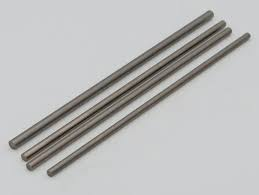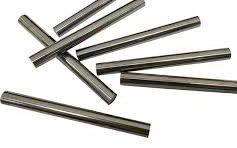# MOLYBDENUM RWMA CALSS 14Molybdenum is not as hard as Class 13 but can be drilled and machined to special contours. With relatively good thermal conductivity, molybdenum has a melting point of 2,623 ºC (4,753 ºF) and has one of the lowest coefficients of thermal expansion among commercially used metals. Suited for cross wire welding of copper and brass, resistance brazing and some upsetting, also welding of braided copper wire to other materials.

 Item Name: Molybdenum, RWMA Class 14, ASTM B387 alloy 360 or 361 Shape: Bar Discs Inserts Plate Rod RWMA (Resistance Welding Manufacturing Alliance) Class: Class 14 Principal Element: Mo Minimum Hardness Rockwell (up to 1" dia.): HRB 85 Minimum Electrical Conductivity (% IACS Min): 30
 ISO Certification: 9001:2015 (Registration # 10002745 QM15 Valid until 2021-08-21) Reports: Certification of Compliance Chemical Analysis Final Inspection Material Test Reports Mechanical and Physical Testing: Elongation Grain Size Hardness Tensile Yield Nondestructive Testing: Chemical Analysis Conductivity Liquid Penetrant Ultrasonic X-Ray
 Alloy Applications Cross Reference Chart C15000 C18150 C18000 C18200 C17510 C17200 C15760 Axial Conductors X Back-up Electrodes X X X X X X X Bushings X X X X X Caps & Shank X X X X X X X Casting Wheels X X X Casting Wheels and Dam Blocks X X X Centrifugal Casting Mold X X X X Circuit Breaker Parts X X X X Collector Connector X X X Collector Housing X X X Connector Bar X X X Connectors X X X X X X Connection Lead Bar X X X Continuous Casting Molds X X X X X X Cross Wire Welding Electrodes & Components X X X X X X X Damper Ring/Finger X X X X Dies & Fixtures X X X X X X Die Casting Pistons X X X Die Casting Plunger Tips X X X Electrode Holders & Adaptors X X X X X X Electrical & Mechanical Power Components X X X X X X X Flash & Butt Welding Dies X X X X X X X Flexible Connector X X X Heat Flux Components X X X X X X Injection Blow Molds & Dies X X X X X Joining Components X X X X X Lead Conductor Bar X X X Motor End Rings X X X X X Neutral Bus Bar X X X Non-Sparking Applications X X X X X Nut & Stud Welding Electrodes X X X X X X Performance Engine Components X X X Permanent Molds X X X X Power Generation Products X X X X Power Semiconductor Bases X X X Resistance Welding Electrodes X X X X X X Rotor Coil Bar X X X Seam Weld Wheels X X X X X X Series Loop Segment X X X Shafts X X X X X X Slip Rings X X X Slot Wedge X X X X Snap Rings X X X Special Nipple X X X Terminal Box Connectors X X X Threaded Electrodes X X X X X X Transmission Devices X X X X Water Clip X X X Wear Plates X Welding Arms X X X X X X Welding Blocks X X X X Welding Connectors X X X X X X Welding Platens X X X X X X